### Home > A2C > Chapter 10 > Lesson 10.2.1 > Problem10-66

10-66.
1. If f (n) = n!, evaluate each of the following ratios. Homework Help ✎

1.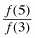2.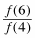3.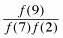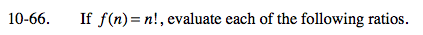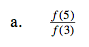$\frac{5!}{3!}=\frac{5\cdot 4\cdot 3\cdot 2}{3\cdot2}$

20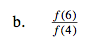See part (a).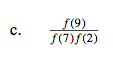See part (a).

36